## Beyond the fourth dimension

``Whithout doubt the authentic type of these figures exists in the mind of God the Creator and shares His eternity''
Johannes Kepler

Visualizing the fourth dimension is hard enough. To go beyond that becomes very difficult indeed. For this reason, we start this by going a bit deeper into some general concepts on polytopes and groups. These allow a deeper understanding of polytopes and their symmetries, and will be very helpful moving further.

### Coxeter-Dynkin diagrams

We present below Zometool models of the Coxeter-Dynkin diagrams for the polytopes shown in this site. In the Zometool system, the yellow struts connect to the triangular holes in the connectors (the balls), they here represent the 3-symmetry branch in the C-D diagrams (which have no attached number because they are so common). The blue struts connect to rectangular holes in the connectors, they represent the ``4'' branches in the C-D diagrams where they appear. The red struts connect to the pentagonal holes in the connectors, they represent the ``5''-branches in the C-D diagrams where they appear. The half-length green struts connect to the pentagonal holes, but they have a twist and are only half length of a regular green, for that reason they symbolize here the ``5/2''-branches in the C-D diagrams where they appear.

The diagrams with only white balls represent a Coxeter group, which is akin to a set of mirrors in a Kaleidoscope. In the case of polyhedra, these can be thought as dividing a spherical surface in a set of identical Schwarz triangles. In the case of polychora, these can be thought as dividing a hyper-spherical surface in a set of identical Goursat tetrahedra. Identical diagrams with only red balls at opposite ends represent dual polytopes.

Regular polytopes and their rectifications can then be thought as reflections of one of the vertices of these triangles/tetrahedra. This is the Kaleidoscopic Wythoff construction. The vertex is indicated by the opposite spherical node being represented by a red ball (in the C-D diagrams, this is represented by circling the node). Note that many polytopes can be represented by multiple C-D diagrams.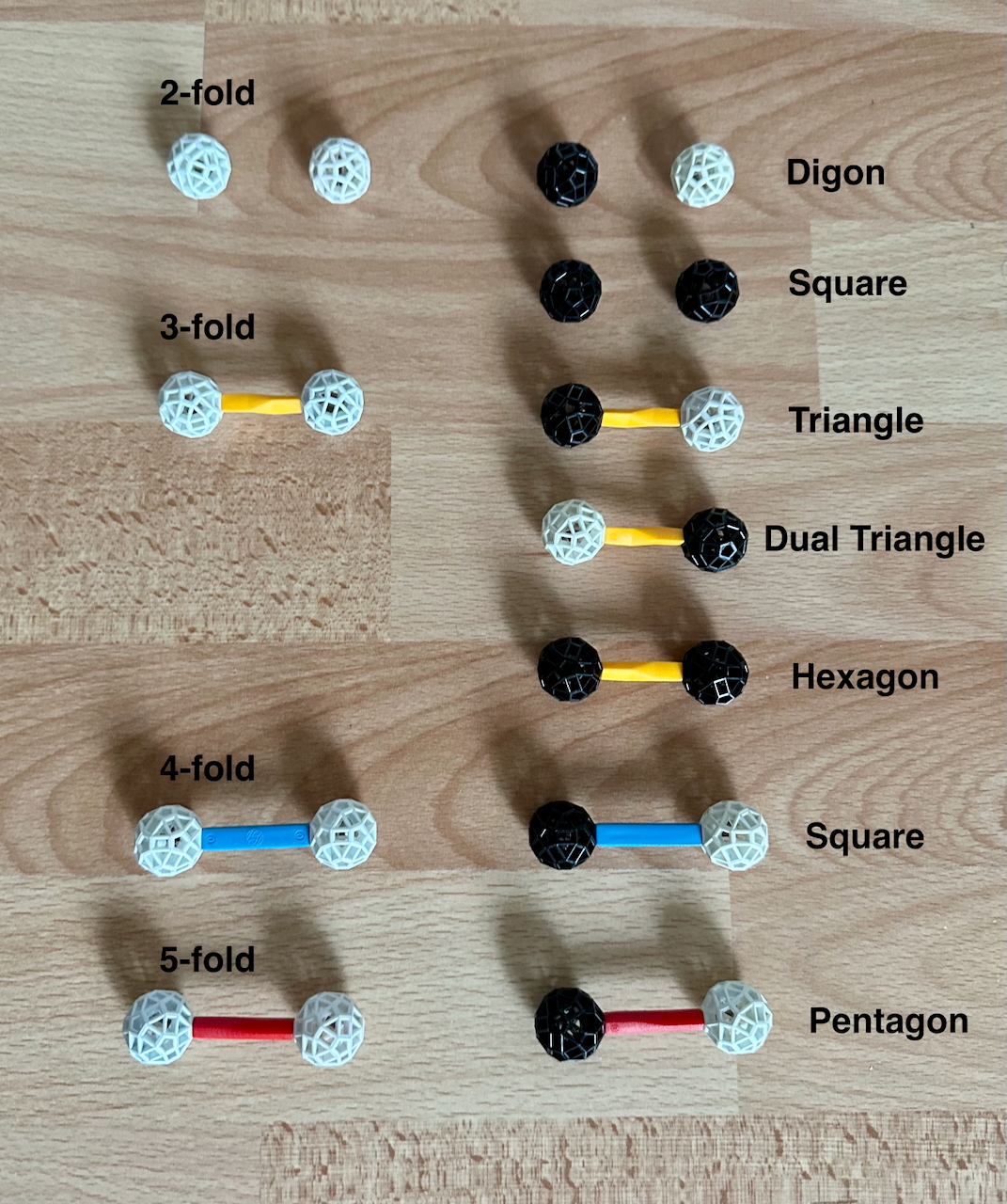Fig. 36: These are the C-D diagrams of the regular polygons used in the polychora in this site, plus their generating groups. All these graphs are left-right symmetric, this means that all regular polygons are self-dual. The number of such diagrams is, of course, infinite.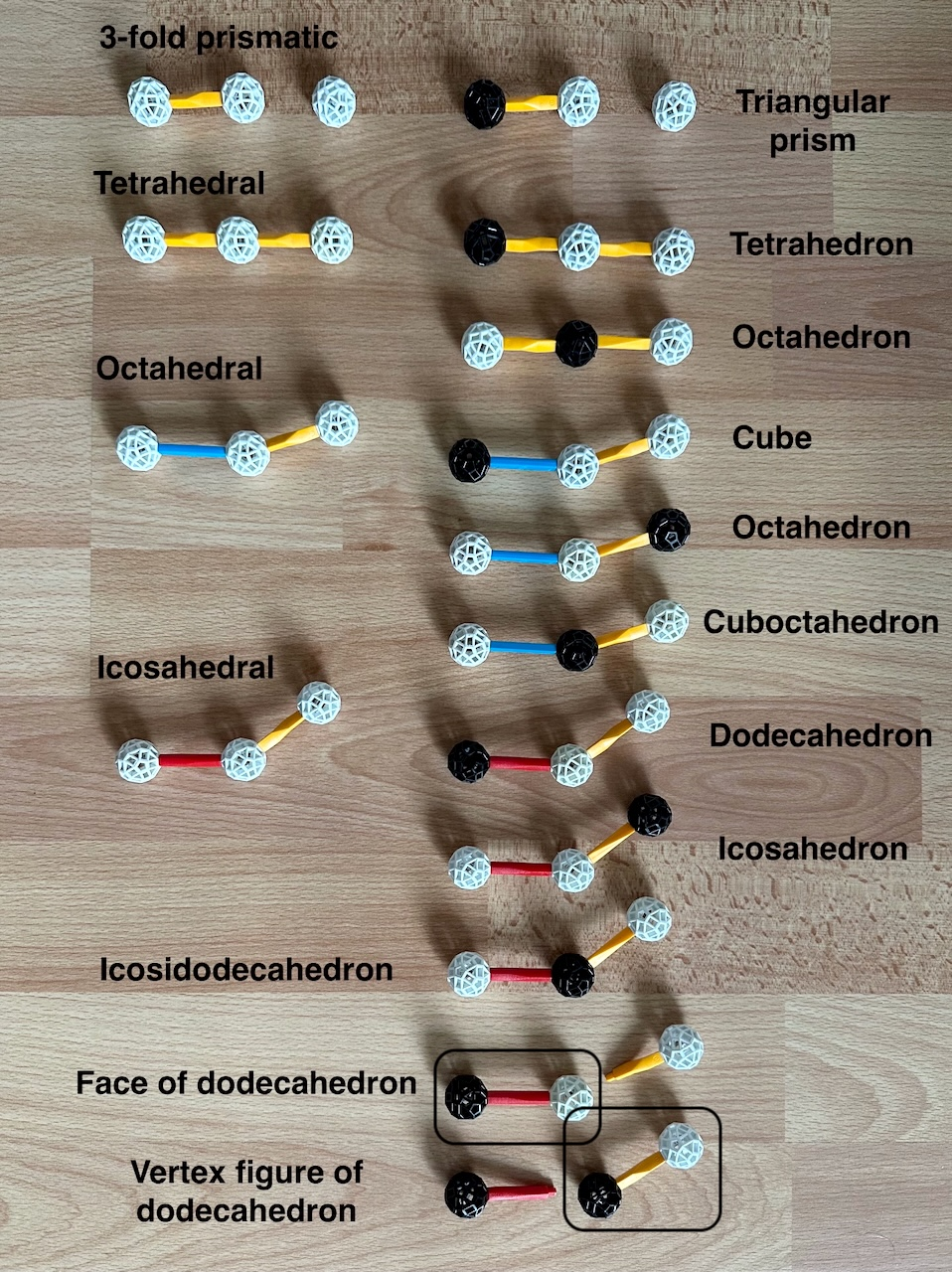Fig. 37: These are the C-D diagrams of the regular and quasi-regular polyhedra in this site, plus their generating groups. On the left column we have the graphs for the groups, in the middle column we have the convex polyhedra, and in the right column we have the star polyhedra.

These diagrams include the polygonal diagrams in Fig. 36, such polygons are thus a part of the polyhedron. For instance, for the Cube, the only polygon graph that can be built with the red ball is the Square.

However, for the Icosidodecahedron, we can form the graphs of the Pentagon and the Equilateral triangle with the red ball (since the graph can be read in both directions); thus the polyhedron is bound by both types of polygons.

Two neighboring white nodes indicate a regular vertex figure: from this, we can see immediately that the vertex figure of the Cube is a regular triangle. The vertex figure of the Cuboctahedron or Icosidodecahedron are more interesting. They are both rectangles. We must imagine here that the diagram forms a loop, with the invisible line connecting the faraway nodes being a ``2-fold'' symmetry axis. This corresponds to the vertex of the Schwarz triangle that is a right angle, such angles are not represented in Coxeter-Dynkin diagrams. The red ball is opposite to this node, this means that to generate the quasi-regular polyhedra we are reflecting the point at the right angle (hence the term ``rectified''). The vertex figure of a point in a 2-fold symmetry axis will therefore be a polygon formed by the two white balls in the circuit, which are connected by this invisible ``2-fold'' line. This has 2-fold symmetry - a Rectangle.

In the polyhedra page, we said that regular and quasi-regular polyhedra share the same Kaleidoscopic Wythoff construction. This fact is evident in the C-D diagrams by the fact that all polyhedron graphs have only one red ball, i.e., they are reflections of vertices of their Schwarz triangles. Other Wythoff constructions, which reflect points in the edges or in the face of the Schwarz triangles to create many uniform polyhedra, would require more than one red ball.

An important point to re-emphasize is that a particular polyhedron (like the Octahedron) can be represented by different diagrams. This is a reflection of the fact that the same polyhedron can be produced from different symmetries. These polyhedra have several other C-D diagrams, we present here those where only one red ball is needed; which directly implies regularity or quasi-regularity.
The A3 graph is symmetric, therefore the Tetrahedron can be generated with the red ball at either end. This means that the Tetrahedron is delf-dual.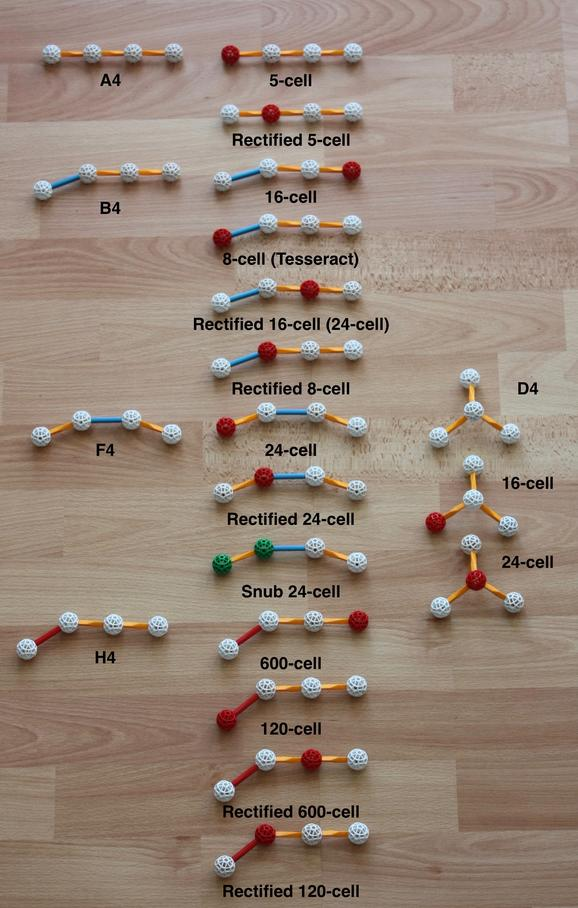Fig. 38: These are the C-D diagrams of the convex polychora presented in the polychora page, plus their generating groups.

On the left we have most of the group graphs. In the middle we have the convex polychora displayed in the polychora page. These include as sub-sets the polyhedral graphs in the middle column of Fig. 37. For instance, in the 16-cell, the only polyhedral graph that can be built with the red ball is the Tetrahedron, therefore the 16-cell only has Tetrahedra, and is therefore regular. In the case of the rectified 5-cell, however, we can build the graphs of the Octahedron and the Tetrahedron from the red ball; this means that that polychoron is bound by both types of polyhedra. Three neighboring white nodes indicate a regular vertex figure (which happens, of course, only for regular polychora), with the equivalent of the red ball next to the actual red ball. Doing this for the 600-cell, for instance, we see that the vertex figure is an Icosahedron. Doing this for a the Rectified 600-cell, we must imagine a 2-fold symmetry line merging the two outer nodes. The white balls then form a polyhedral figure with 5-fold symmetry along one direction and 2-fold symmetry along the other - a (non-uniform) Pentagonal prism.

Most, but not all, of the polytope graphs presented here (and corresponding polytopes in this site) have the same Wythoff construction, this is indicated in the graphs by the single red ball (this indicates reflection of a vertex of the Goursat tetrahedron). The exceptions are the diminished 600-cells: the Snub 24-cell (see Fig. 17), which has two green balls (indicating rotational but not reflexive symmetry) and the Grand antiprism. The latter does not appear in this Figure because (as mentioned in the caption to Fig. 22) it has no Wythoff construction.

Many other convex uniform polychora are possible with the use of more red balls, but building all of them requires many parts, as one can see in David Richter's H4 page. Just one example here: the truncated 600-cell. Its graph has two red balls, indicating that the vertex is a reflection of a point on an edge of the Goursat Tetrahedron.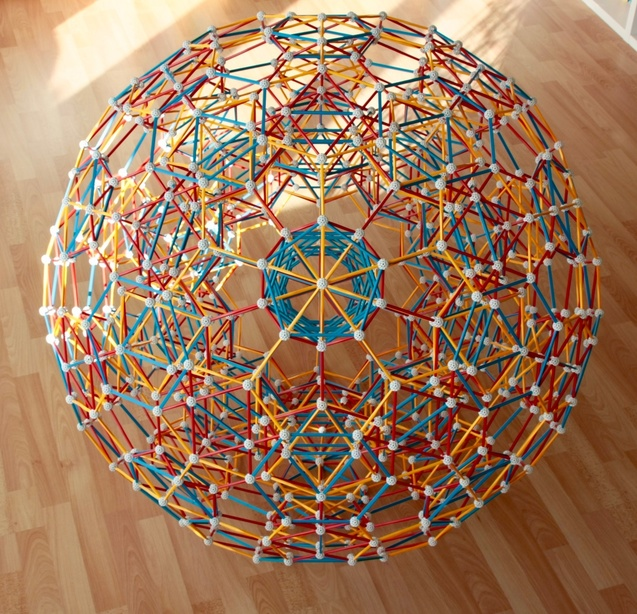Fig. 39: The truncated 600-cell, one of the ``Archimedean'' polychora. This Zometool model has a lot of parts, and it is quite flimsy.

Studying these diagrams, we can see that the process of rectification of a regular polychoron with X-type of cell and Y-type of vertex figure generates a new polychoron for which the cells are rectified Xs (as many as the previous number of cells) and Ys (as many as the previous number of vertices). The number of vertices will be the same as the number of edges in the polychoron before.

Again, almost all of these polychora can be represented by multiple C-D diagrams; those presented here are those that require only one red ball. Note that the 24-cell is represented by 3 different diagrams in the figure, and the 16-cell by two. Some of these extra diagrams appear in the right column, we have, on top, the Demi-hypercubic group D4.
In 4 dimensions, this type of symmetry does not yet produce new polytopes, this starts happening only in 5 dimensions. However, it has some interesting consequences, one of them being Triality.

As mentioned in the polychoron compounds page, in the case of duality in 3 dimensions, one can, by the definition of the meaning of duality, scale two concentric dual polyhedra in such a way that each edge from a polyhedron meets another (perpendicular) edge from the dual polyhedron at a point that is in the middle of both (the midpoint). Those two edges form a plane that is perpendicular to a third line joining the midpoint to the center of those polyhedra. Rectifying those two dual polyhedra results in the same rectified polyhedron.
In the case of Triality in 4 dimensions, the situation is very similar, except that the perpendicular edges of three concentric ``trial'' polychora (16-cells for the only regular case) meet at their midpoints. These edges are in a 3-D ``plane'' that is itself perpendicular to a fourth direction, the line joining those midpoints to the centers of the trial polychora. Rectifying all three polychora results in the same rectified polychoron, the regular case being the 24-cell.
This possibility is a consequence of the symmetry of the D4 graph. In this graph, we can imagine all outer nodes to be connected by an invisible ``2-fold'' line, this is what gives us this possibility of having three perpendicular directions intersecting along a direction that is orthogonal to the central direction.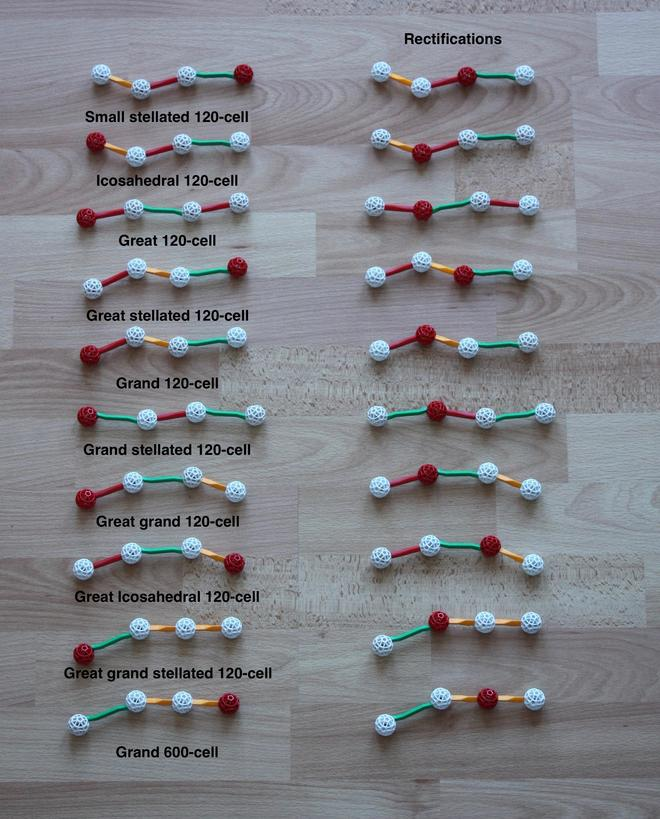Fig. 40: These are the C-D diagrams of the regular star polychora presented in the polychora page (on the left column), plus those of their rectifications (on the right column). I have built models for all polychora on the left, but not for their rectifications (for lack of space, time and money...), except for one or two (see below).

These also include the star polyhedra diagrams in the right column of Fig. 37. From these diagrams, we can see, for instance, that the Small stellated 120-cell is bound by Small stellated dodecahedron cells (the only polyhedral graph that can be built from the red ball) and that this has a Dodecahedral vertex figure. Thus its dual, the Icosahedral 120-cell, has Icosahedra as cells, and Great dodecahedra as vertex figures. We can also see that, following the logic of rectification, the Rectified small stellated 120-cell has Dodecadodecahedra and Dodecahedra as cells, and that the Rectified icosahedral 120-cell has Icosidodecahedra and Great dodecahedra as cells.

Note that the edges of the latter rectified polychoron are identical to those of the Rectified 600-cell; thus both can be represented by the same Zometool model. The identical rectification is a consequence of the conservation laws in Fig. 26c: the rectifications of two polytopes that share the same edge arrangement will necessarily share the same vertex arrangement, since the vertex of the rectification is the middle point of the edge of the original polytope. The rectifications of two polytopes that additionally share the same face arrangement (like the 600-cell and the Icosahedral 120-cell) will necessarily share the same edge arrangement.
These graphs also tell us something interesting: the rectification of the Grand 600-cell is, like the rectification of the 600-cell, a semi-regular polychoron, the only such case among these ten rectifications. The cells are Great icosahedra and Octahedra. By the rule we have mentioned in the previous paragraph, that Zometool model will be the same as the model of the Rectified great Icosahedral 120-cell.

The symmetry of graphs for the Great 120-cell and the Grand-stellated 120-cell indicates that these are self-dual.

### Now, beyond the fourth dimension

What happens at dimensions higher than 4?

The only regular polytopes are the Simplexes (generated by the An groups, where n is the dimension number), the Hypercubes and their duals, the Orthoplexes, or cross polytopes, generated by the Bn groups. These An and Bn group families are infinite, extending to any number of dimensions. Apart from them, there are no equivalents of Icosahedra, there are no regular star polyhedra either.

In this way, one comes to appreciate the fact that a few of the symmetries shown above, like the Icosahedral (H3), Hexacosichoric (H4) and Icositetrachoric (F4) symmetries, are exceptional objects - special cases that happen only rarely. They are among the rare wonders of mathematics.

There are other interesting symmetries in higher dimensions. There is one more infinite family of uniform polytopes that has been thus far hidden: the Demi-hypercubes, generated by the Dn group family. These are obtained by removing alternate vertices of a Hypercube. Although they generate interesting phenomena in 4 dimensions, like the aforementioned triality, this family is thus far hidden because all polyhedra and polychora built with the symmetry of the Demi-cube and Demi-tesseract already exist as members of other families: the Demi-cube is the Tetrahedron and the Demi-tesseract is the 16-cell. It is only in 5 dimensions that it starts generating new polytopes (through the Wythoff construction) that are unique to it. One of these is the semi-regular Demipenteract, see Fig. 41. As we can see from the Figure, this has either 16-cells or 5-cells as 4-faces, which are the polychoron diagrams that we can form including the red ball.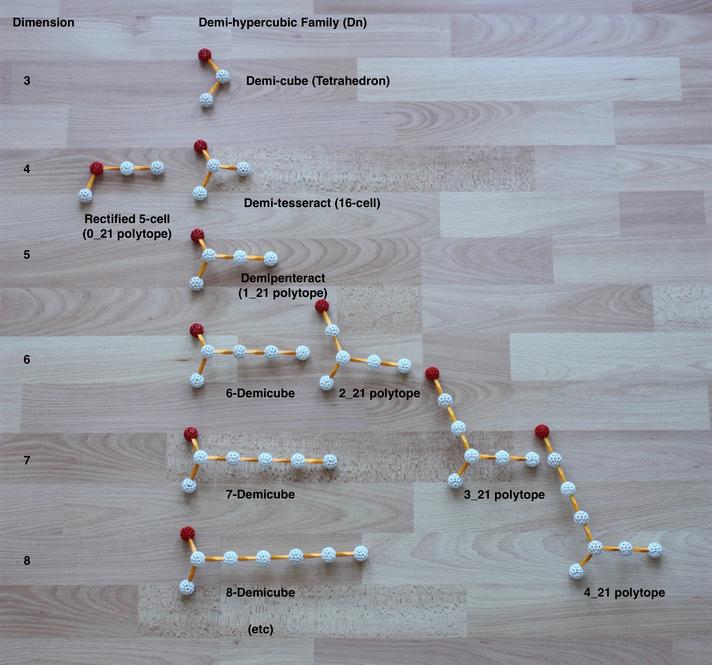Fig. 41: C-D diagrams for some of the higher-dimensional polytopes. In the center we see the infinite Demi-hypercubic family. All polytopes of this family in 3 and 4 dimensions are also generated by the B3 and B4 groups. In 5 dimensions, the D5 family starts generating unique symmetries that are not present in the An or Bn families.

We also see the k21 family evolving along the diagonal. This ends at 8 dimensions, with the 421 polytope. The next member of the family is an infinite tesselation filling 8-dimensional space.

The Demipenteract is interesting because, as we can see in Fig. 41, it also belongs to the finite semi-regular k21 polytope family (the name comes from the length of the branches in the C-D diagrams, with the k indicating the length of the branch where the red ball is). In this family the facets of each polytope are Simplexes and Orthoplexes and the vertex figure given by the (k-1)21 polytope below it. In that family the Demipenteract is the 121 polytope. Its vertex figure is the 021 polytope; as mentioned in the polychora page, this is the rectified 5-cell.

In 6 dimensions, the k21 family starts generating polytopes with symmetries unique to it. Of particular interest is the 221 semi-regular polytope. This and 38 other uniform polytopes are constructed within the symmetry of the E6 group.

In seven dimensions, the semi-regular 321 polytope and 126 other uniform polytopes are constructed within the symmetry of the E7 group.

Finally, in eight dimensions, the semi-regular 421 polytope (see Figures 42a, b and 43 below) and 254 other uniform polytopes are constructed within the symmetry of the E8 group. The largest of these, the Omni-truncated 421 polytope, has 696,729,600 vertices, the number of symmetries of this group.

That's it! The family ends, because its next member (still in eight dimensions!) is the infinite 521 honeycomb. Its vertex figure is the E8 polytope and its vertex arrangement is the E8 lattice; this provides the most compact sphere packing in 8 dimensions. In 8-dimensional space, each hypersphere can ``kiss'' 240 other hyperspheres (note that 240 is the number of vertices of the 421 polytope).

Thus, one comes to the realization that the E6, E7 and E8 are also exceptional objects - rare jewels among the infinite geometric treasures we have just contemplated.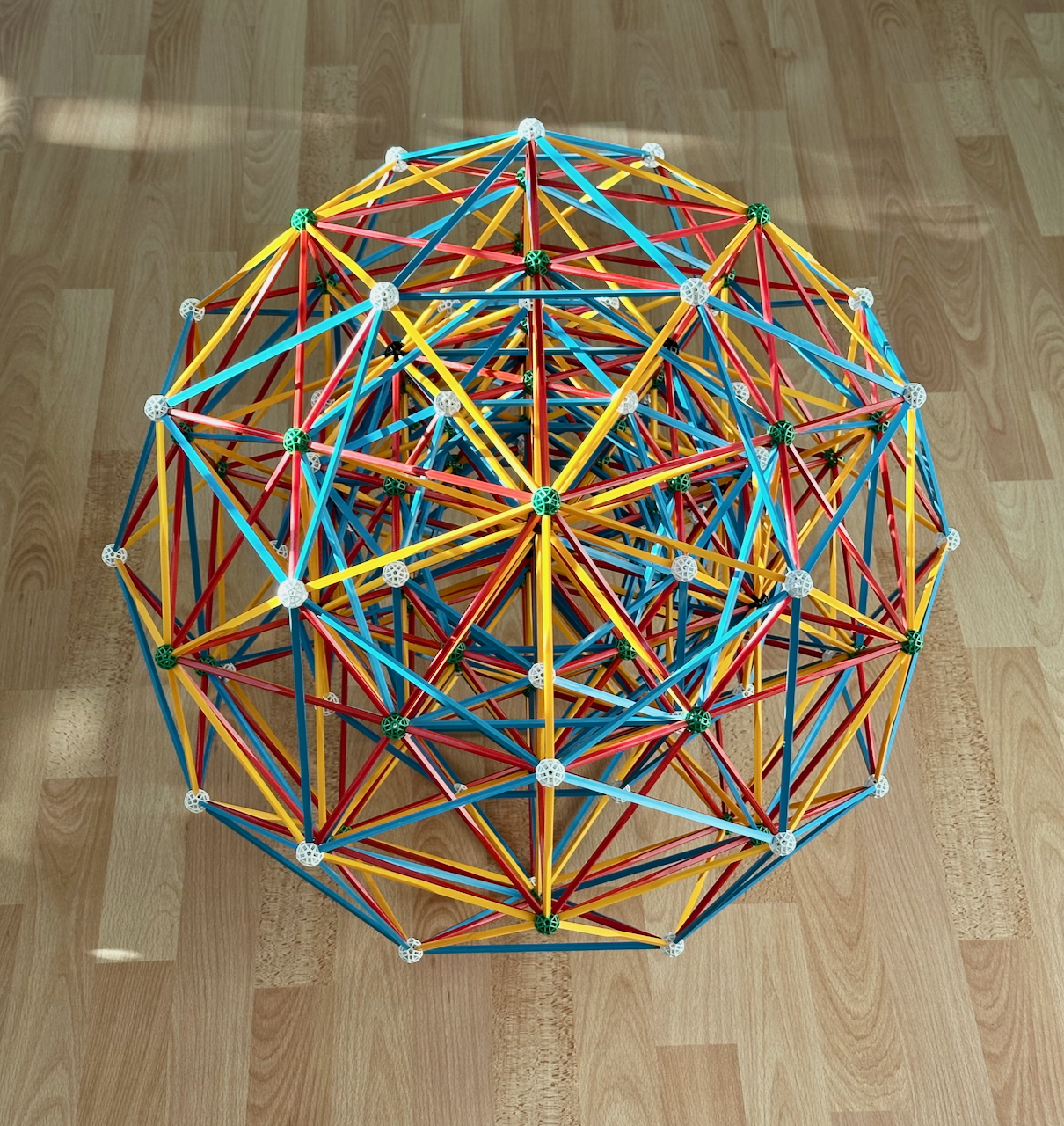Fig. 42a: A Zometool model of the 421 polytope. The 240 vertices project here as the vertices of two concentric 600-cells, with one of them being larger than the other by a factor of φ. It is not possible to project all the edges into a 3-D Zometool model, so we must choose a sub-set. In this case, we chose the edges of the projections of the two concentric 600-cells, exactly as in David Richter's model. Here we can see that projecting higher-dimensional figures in three dimensions leads to a concentration of vertices and edges near the center.
This model required many old very long struts, no longer manufactured by Zometool: 120 very long yellows, 120 very long blues, and 60 very long reds. Alternatively, the model can be made on a smaller scale.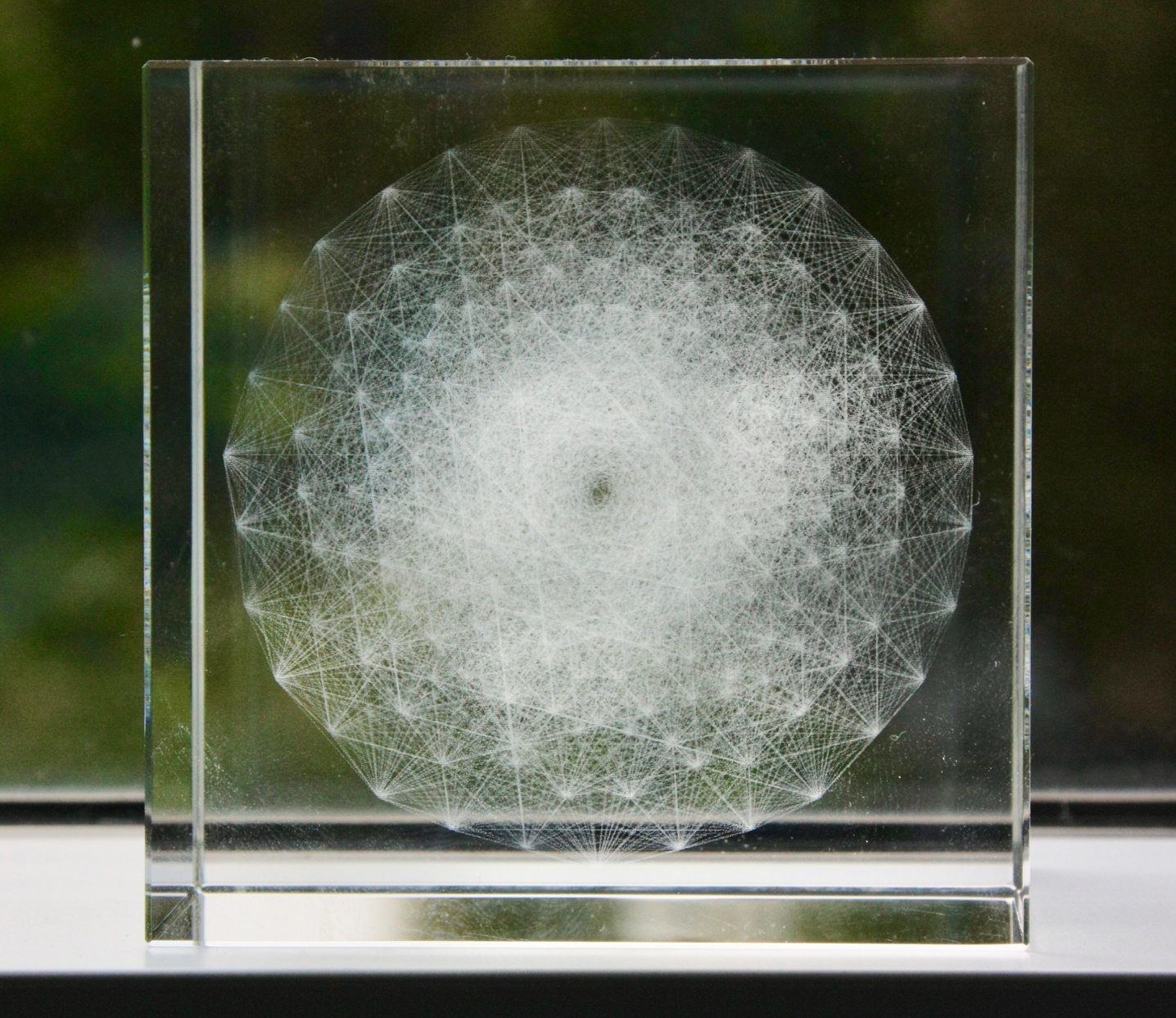Fig. 42b: An orthographic projection of the 421 polytope in 3-D space, now with all the edges represented. This model is laser-engraved in glass.

This was a present given to me by one of my students, Jose Martinez.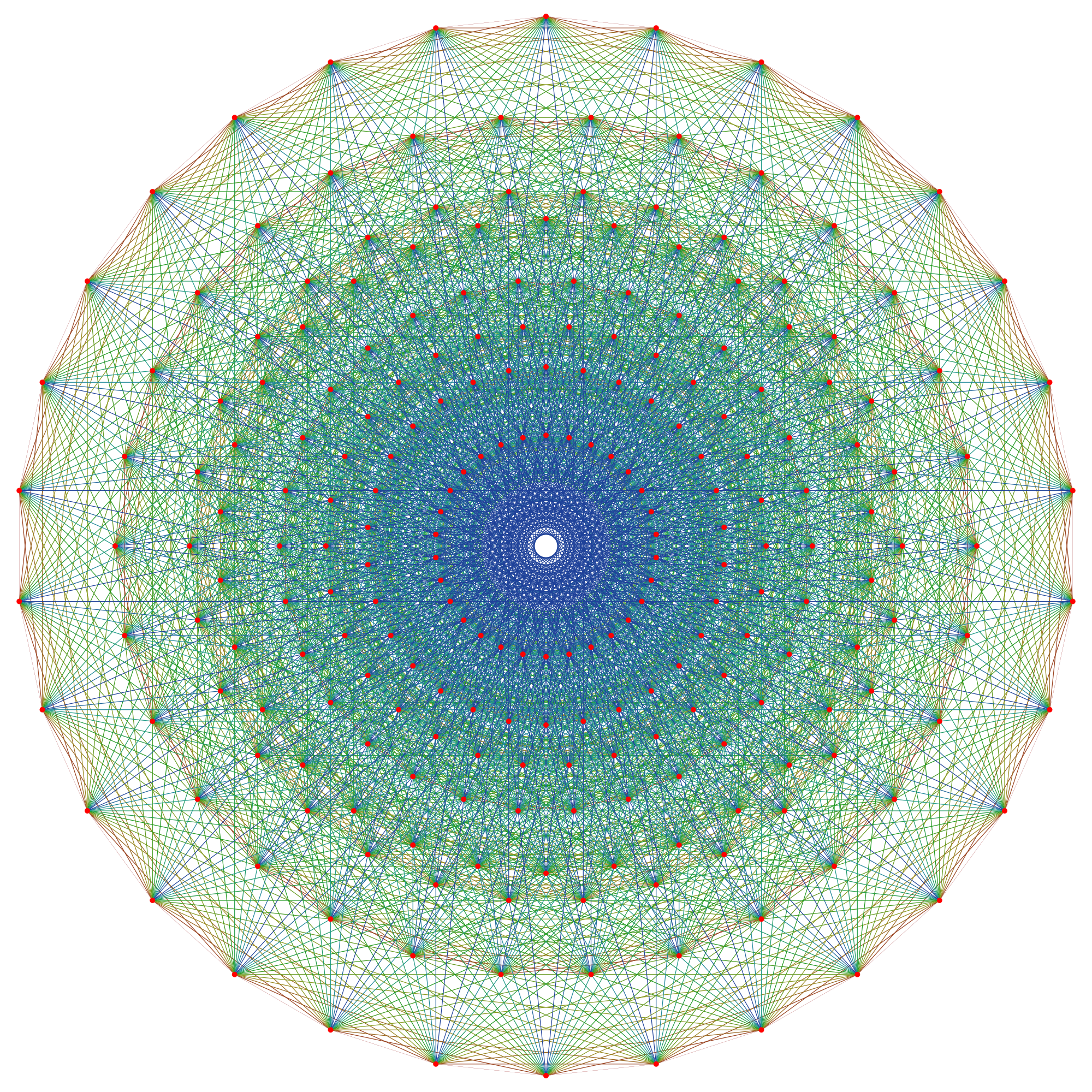Fig. 43: Print on my door: The orthographic projection of the 8-dimensional 421 polytope onto the Coxeter plane where its projection has maximal symmetry. This 2-D projection highlights even more the concentration of vertices towards the center, As its 240 vertices represent the root vectors of the simple Lie group E8, the polytope is sometimes referred to as the ``E8 polytope''. Using a complex number coordinate system, it can also be constructed as a 4-dimensional regular complex polytope known as the Witting polytope.
Image by Claudio Rocchini (Own work), via Wikimedia Commons.

That's not the end of the fun, though. That has just started...

Consider the 24-dimensional Leech Lattice, where each sphere ``kisses'' 196560 neighbors, and provides the most compact sphere packing in that dimension! The number of symmetries in this lattice is:

8 315 553 613 086 720 000.

Or consider the Monster group, which can be represented by a polytope in 196883 dimensions! The number of its symmetries is:

808 017 424 794 512 875 886 459 904 961 710 757 005 754 368 000 000 000,

which is about 6000 times the number of atoms on Earth. As John H. Conway has said, we've only started scratching the surface of the laws of symmetry!

Next: References